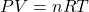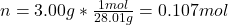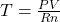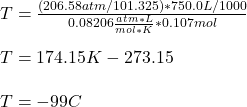A sample of nitrogen gas in a 750.0 mL sealed flask exerts a pressure of 206.58 kPa. Calculate the temperature of the gas if the flask conta

Question

A sample of nitrogen gas in a 750.0 mL sealed flask exerts a pressure of 206.58 kPa. Calculate the temperature of the gas if the flask contains 3.00 grams of gas.

in progress 0
5 months 2021-08-11T10:15:08+00:00 1 Answers 20 views 0

T= -99 °C.

Explanation:

Hello there!

In this case, according to the given description of the problem, it is possible for us to analyze this problem via the ideal gas equation:However, we first calculate the moles in 3.00 grams of nitrogen gas (28.01 g/mol):Next, we solve for the temperature as shown below:Next, we convert the given pressure and volume to atm and L to obtain:Regards!